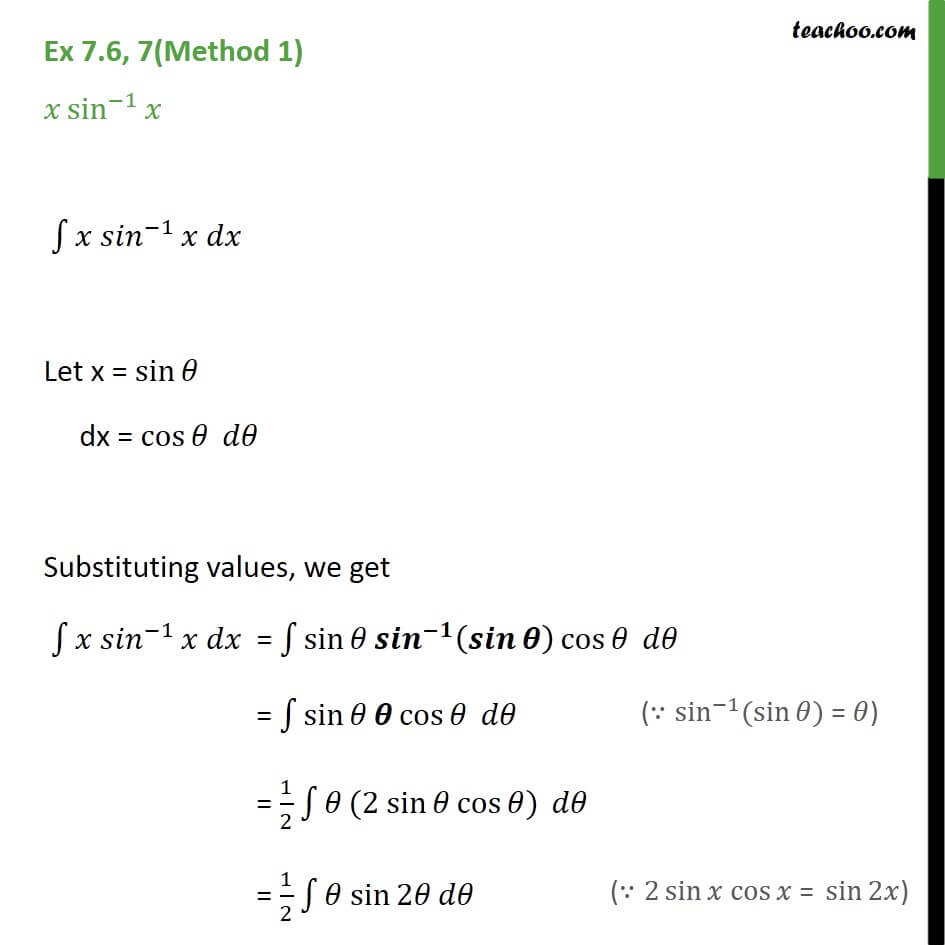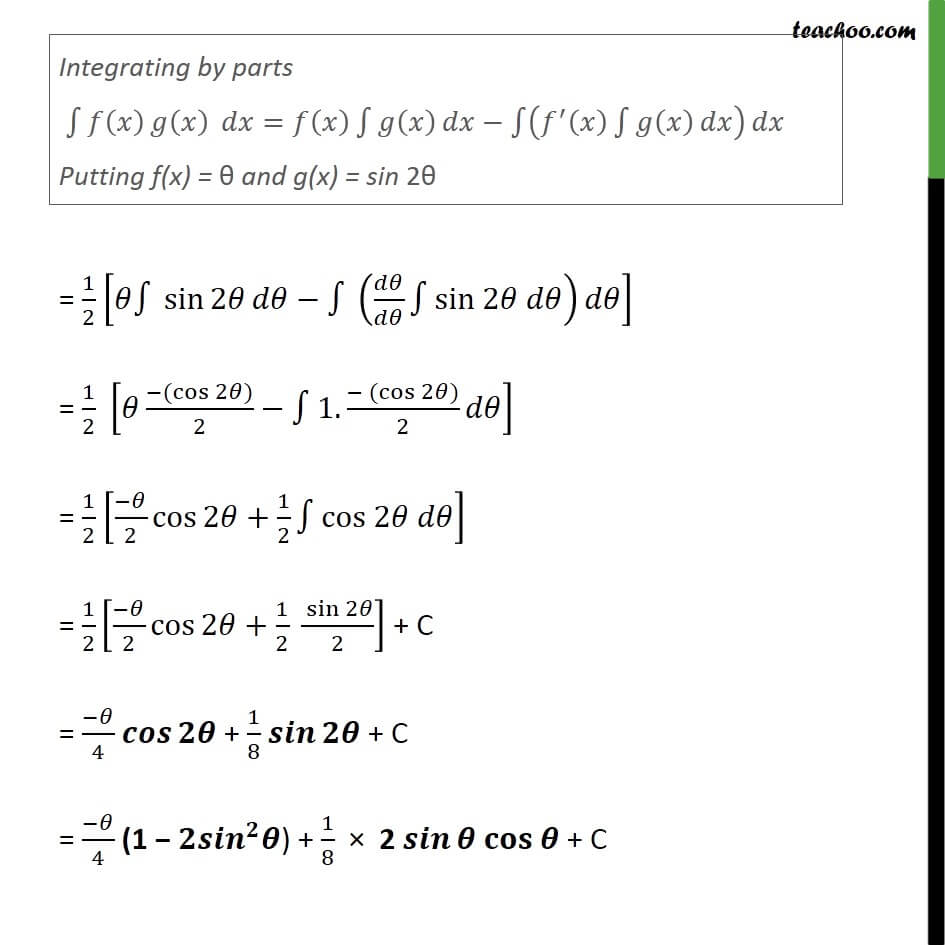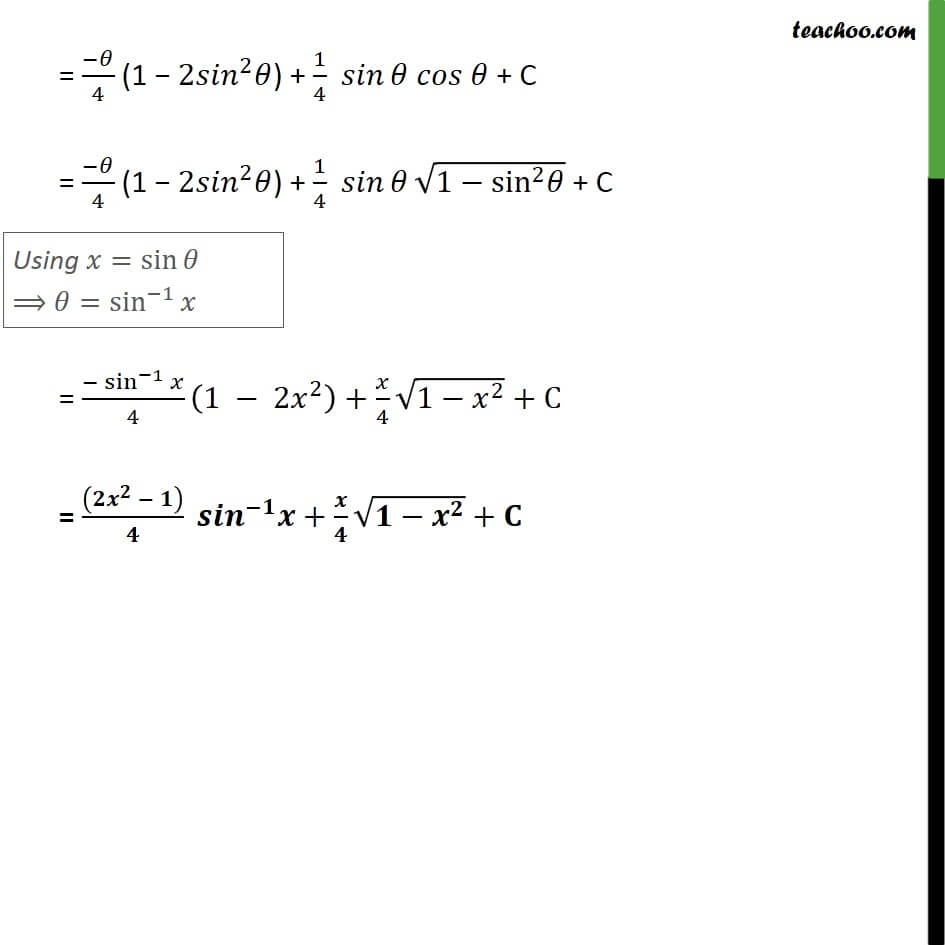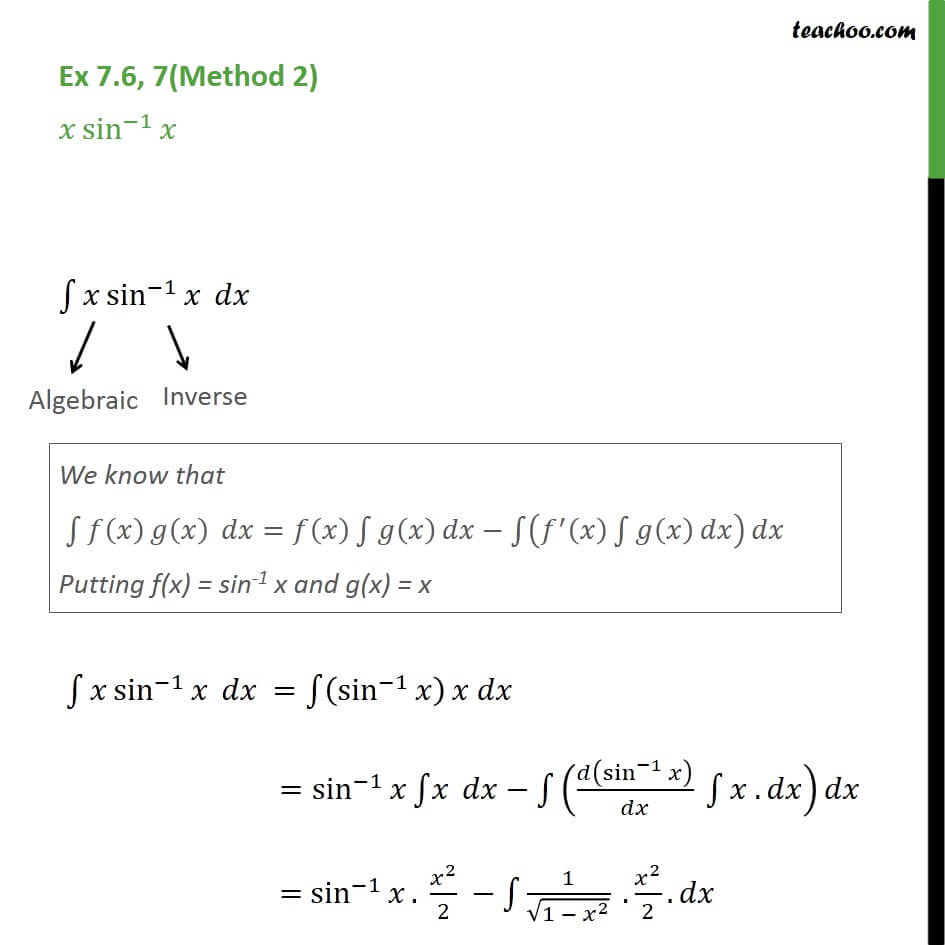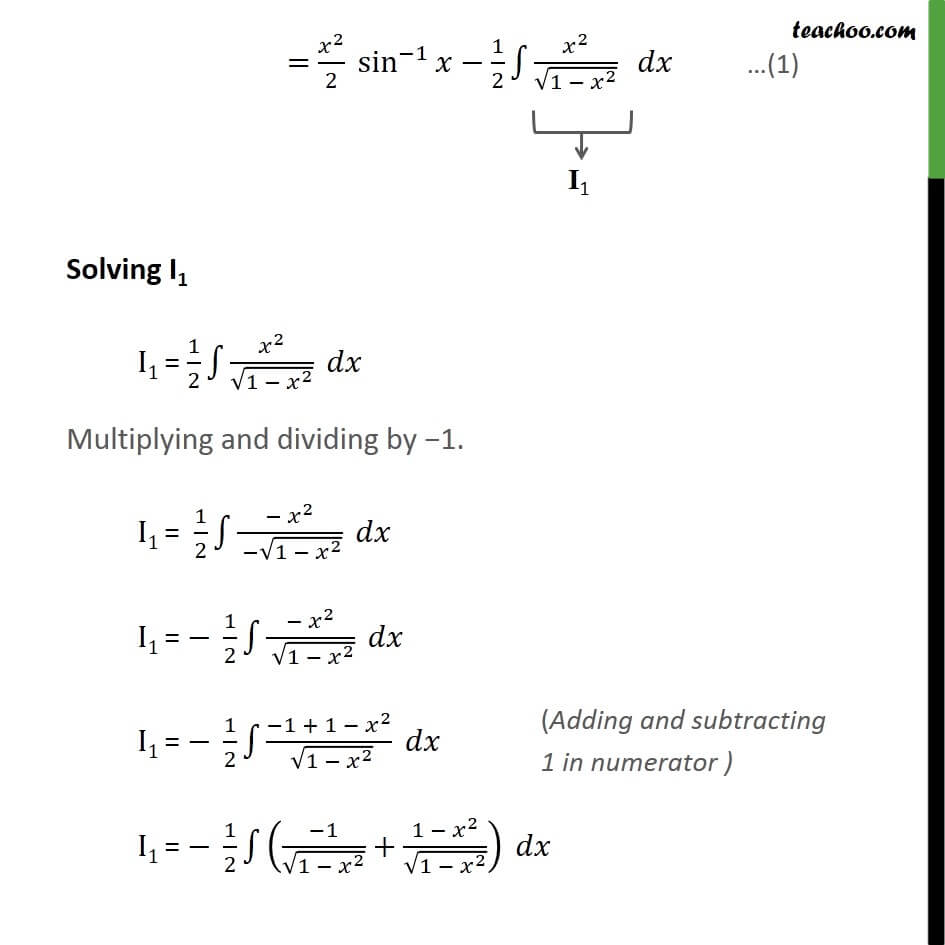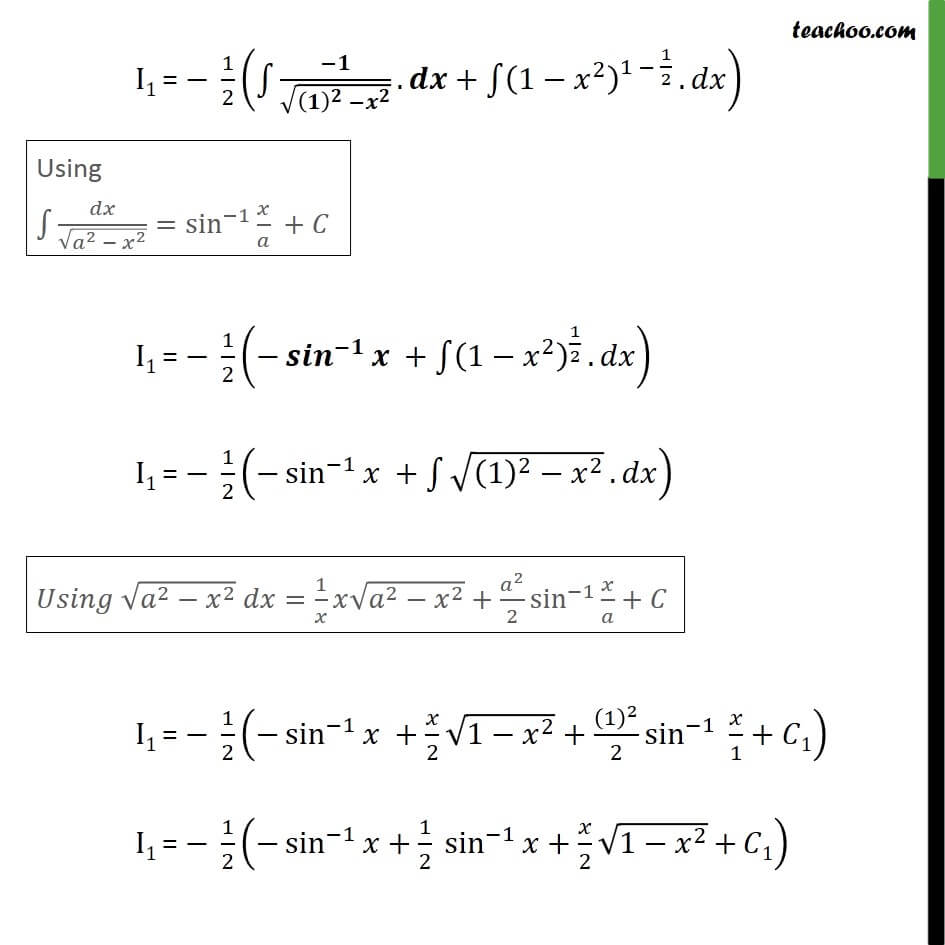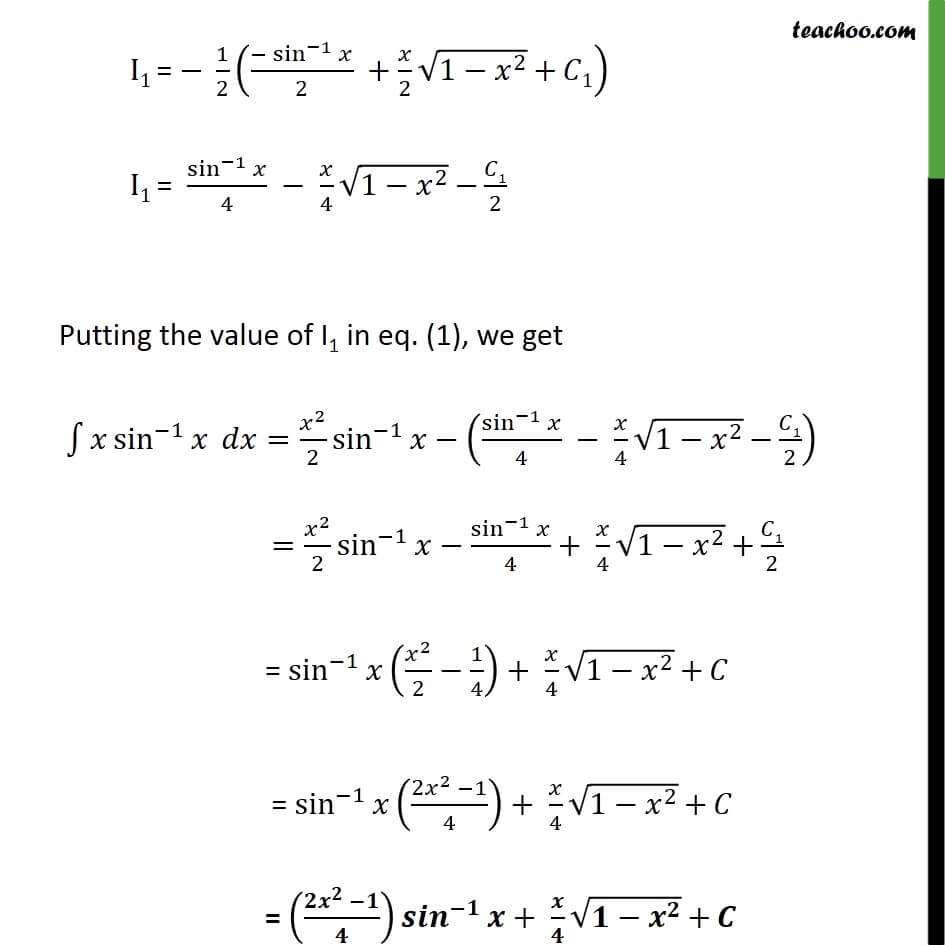1. Chapter 7 Class 12 Integrals
2. Serial order wise
3. Ex 7.6

Transcript

Ex 7.6, 7(Method 1) sin^( 1) 1 ^( 1) Let x = sin dx = cos Substituting values, we get 1 ^( 1) = 1 sin ^( ) ( ) cos " " = 1 sin cos " " = 1/2 1 (2 sin cos ) " " = 1/2 1 sin 2 = 1/2 [ sin 2 ( / sin 2 ) ] = 1/2 [ ( (cos 2 ))/2 1.( (cos 2 ))/2 ] = 1/2 [( )/2 cos 2 +1/2 cos 2 ] = 1/2 [( )/2 cos 2 +1/2 ( sin 2 )/2] + C = ( )/4 + 1/8 + C = ( )/4 (1 ^ ) + 1/8 2 + C = ( )/4 (1 2 ^2 ) + 1/4 + C = ( )/4 (1 2 ^2 ) + 1/4 (1 sin^2 ) + C = ( sin^( 1) )/4 (1 2 ^2 )+ /4 (1 ^2 )+C = (( ^ ))/ ^( ) + / ( ^ )+ Ex 7.6, 7(Method 2) sin^( 1) 1 sin^( 1) 1 sin^( 1) " "= 1 (sin^( 1) ) =sin^( 1) 1 1 ( (sin^( 1) )/ 1 . ) = sin^( 1) . ^2/2 1 1/ (1 ^2 ) . ^2/2. = ^2/2 sin^( 1) 1/2 1 ^2/ (1 ^2 ) Solving I1 I1 = 1/2 1 ^2/ (1 ^2 ) Multiplying and dividing by 1. I1 = 1/2 1 ^2/( (1 ^2 )) I1 = 1/2 1 ^2/( (1 ^2 )) I1 = 1/2 1 1 + 1 ^2/( (1 ^2 )) I1 = 1/2 1 (( 1)/ (1 ^2 )+(1 ^2)/ (1 ^2 )) I1 = 1/2 (( 1)/((1)^2 ^2 ). + 1 (1 ^2 )^(1 1/2) . ) I1 = 1/2 ( sin^( 1) + 1 (1 ^2 )^(1/2) . ) I1 = 1/2 ( sin^( 1) + 1 ((1)^2 ^2 ). ) I1 = 1/2 ( sin^( 1) + /2 (1 ^2 )+(1)^2/2 sin^( 1) /1 + 1) I1 = 1/2 ( sin^( 1) +1/2 sin ^( 1) + /2 (1 ^2 ) + 1) I1 = 1/2 (( sin^( 1) )/2 + /2 (1 ^2 ) + 1) I1 = sin^( 1) /4 /4 (1 ^2 ) 1/2 Putting the value of I1 in eq. (1), we get 1 sin^( 1) = ^2/2 sin^( 1) (sin^( 1) /4 /4 (1 ^2 ) 1/2) = ^2/2 sin^( 1) sin^( 1) /4+ /4 (1 ^2 ) + 1/2 = sin^( 1) ( ^2/2 1/4)+ /4 (1 ^2 ) + = sin^( 1) (( 2 ^2 1)/4)+ /4 (1 ^2 ) + = (( ^ )/ ) ^( ) + / ( ^ ) +

Ex 7.6

Chapter 7 Class 12 Integrals
Serial order wise